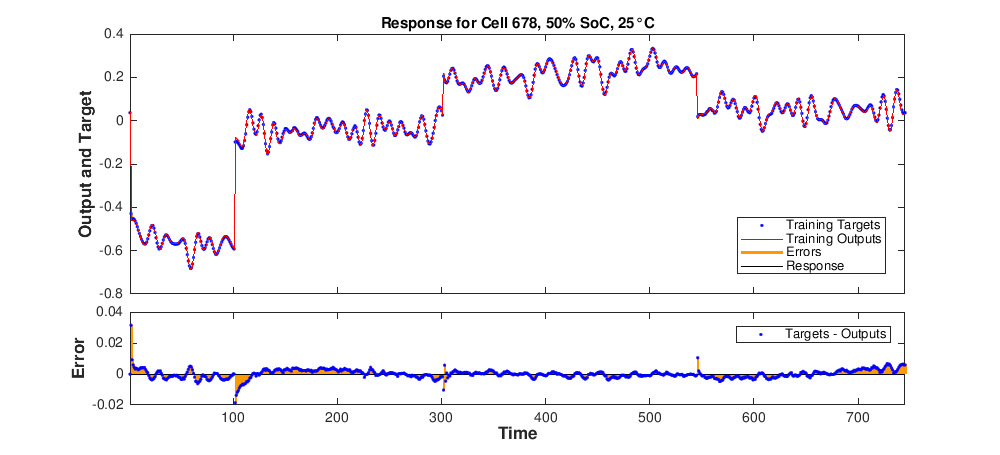# Neural Networks

Neural Networks are a useful tool for system identification and for the study of non-linearity and temperature/Soc dependence in the li-ion battery model. They can be defined as parallel distributed processors made up of simple units (called neurons). The knowledge is acquired by the network through a training process and stored through the interneuron connection strengths, called synaptic weights, which are determined by minimising the loss function. So, we can characterise neural networks by two main ingredients: the network structure and the training algorithm.

Considering the results obtained using subspace methods and non-linear arguments, a good choice for the neural network architecture is a two-layer recurrent (ie. with feedback connections) network with linear and sigmoid activation functions.The behaviour of this neural network can be described by the system of equations$\begin{cases} x(k)=\mathrm{tansig}(a_1 x(k-1)+b_1 i(k-1)+\alpha_1)\\ y(k) = a_2 y(k-1)+b_2 i(k-1)+\alpha_2\\ u(k)=c_1 x(k)+c_2y(k)+d i(k) , \end{cases}$

where$\mathrm{tansig}(n):=2/(1+\exp(-2n))-1$, which is a good approximation of the sigmoid function used by Dhammika Widanage et al. Observe also that the system of equations is similar to the system used for subspace methods, with the addition of a further (non-linear) hidden variable.

Regarding the training algorithm, a good choice is to use the Levenberg-Marquardt back-propagation algorithm used for minimising the mean least squares, in order to guarantee both stability and a quick convergence.

The benefits of using neural networks are several:

• They provide an accurate method for approximate the current-voltage model, and with a low computational cost;
• The use of non-linear functions in the network architecture produces good results also in the case of low SoCs and Temperatures;
• They have a good reproducibility: applying the trained neural network model to a drive-cycle current profile, we obtain a good approximation of the drive-cycle model, provided the network was trained using pulse-multisines with similar SoC and Temperatures;
• Lastly, training neural networks for different SoCs and Temperatures and looking at the network weights for the different parameters allow us to deepen the relation between the estimated model and the SoC and Temperature parameters.